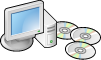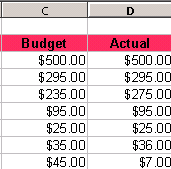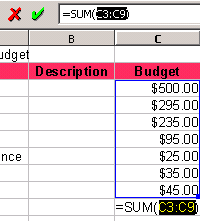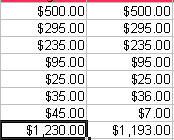# OpenOffice: Calc - Create a Sum

by Mike Weber

Creating a sum of numbers is a basic skill that every user will need to be able to perform. In OpenOffice Calc the procedure is somewhat different than in Microsoft Excel.

Create a list of numbers and place them in cells. For this example we have created a busget and a lis tof expenses in cells C3 to C9. Now, create a sum of all expenses, by clicking on the cell just below the numbers in column C and clicking the sum button on the Formula Bar.You will see the formula =sum(C3:C9) which adds the numbers in cells C3 to C9, giving you a total. You can modify the formula in the Formula Bar window if you want to make changes.Once you see the formula (=SUM(C3:C9) you can hit enter and the sum will be created. An alternative is to click on the green check mark in the formaul bar which will do the same thing.Microsoft,Excel and Microsoft Office are registered trademarks of Microsoft Inc.